Search by Topic

Resources tagged with Maths Supporting SET similar to Root Hunter:

Filter by: Content type:
Age range:
Challenge level:

There are 93 results

Broad Topics > Applications > Maths Supporting SET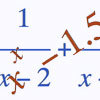Root Hunter

Age 16 to 18 Challenge Level:

In this short problem, try to find the location of the roots of some unusual functions by finding where they change sign.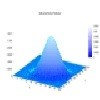Pdf Matcher

Age 16 to 18 Challenge Level:

Which pdfs match the curves?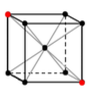Crystal Symmetry

Age 16 to 18 Challenge Level:

Use vectors and matrices to explore the symmetries of crystals.Nine Eigen

Age 16 to 18 Challenge Level:

Explore how matrices can fix vectors and vector directions.Square Pair

Age 16 to 18 Challenge Level:

Explore the shape of a square after it is transformed by the action of a matrix.Transformations for 10

Age 16 to 18 Challenge Level:

Explore the properties of matrix transformations with these 10 stimulating questions.Counting Dolphins

Age 14 to 16 Challenge Level:

How would you go about estimating populations of dolphins?Debt Race

Age 16 to 18 Challenge Level:

Who will be the first investor to pay off their debt?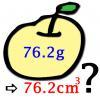More or Less?

Age 14 to 16 Challenge Level:

Are these estimates of physical quantities accurate?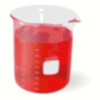Brimful 2

Age 16 to 18 Challenge Level:

Which of these infinitely deep vessels will eventually full up?Aim High

Age 16 to 18 Challenge Level:

How do you choose your planting levels to minimise the total loss at harvest time?Vector Walk

Age 14 to 18 Challenge Level:

Starting with two basic vector steps, which destinations can you reach on a vector walk?Stirling Work

Age 16 to 18 Challenge Level:

See how enormously large quantities can cancel out to give a good approximation to the factorial function.Fix Me or Crush Me

Age 16 to 18 Challenge Level:

Can you make matrices which will fix one lucky vector and crush another to zero?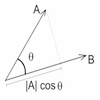Cross with the Scalar Product

Age 16 to 18 Challenge Level:

Explore the meaning of the scalar and vector cross products and see how the two are related.Polygon Walk

Age 16 to 18 Challenge Level:

Go on a vector walk and determine which points on the walk are closest to the origin.Matrix Meaning

Age 16 to 18 Challenge Level:

Explore the meaning behind the algebra and geometry of matrices with these 10 individual problems.Big and Small Numbers in Biology

Age 14 to 16 Challenge Level:

Work with numbers big and small to estimate and calulate various quantities in biological contexts.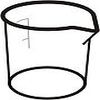Brimful

Age 16 to 18 Challenge Level:

Can you find the volumes of the mathematical vessels?Biology Measurement Challenge

Age 14 to 16 Challenge Level:

Analyse these beautiful biological images and attempt to rank them in size order.Perspective Drawing

Age 11 to 16 Challenge Level:

Explore the properties of perspective drawing.Scientific Curves

Age 16 to 18 Challenge Level:

Can you sketch these difficult curves, which have uses in mathematical modelling?Equation Matcher

Age 16 to 18 Challenge Level:

Can you match these equations to these graphs?Production Equation

Age 16 to 18 Challenge Level:

Each week a company produces X units and sells p per cent of its stock. How should the company plan its warehouse space?Dangerous Driver?

Age 16 to 18 Challenge Level:

Was it possible that this dangerous driving penalty was issued in error?Integration Matcher

Age 16 to 18 Challenge Level:

Can you match the charts of these functions to the charts of their integrals?Global Warming

Age 14 to 16 Challenge Level:

How much energy has gone into warming the planet?Big and Small Numbers in the Living World

Age 11 to 16 Challenge Level:

Work with numbers big and small to estimate and calculate various quantities in biological contexts.Differential Equation Matcher

Age 16 to 18 Challenge Level:

Match the descriptions of physical processes to these differential equations.The Monte Carlo Method

Age 16 to 18 Challenge Level:

Estimate areas using random gridsPdf Stories

Age 16 to 18 Challenge Level:

Invent scenarios which would give rise to these probability density functions.Quorum-sensing

Age 16 to 18 Short Challenge Level:

This problem explores the biology behind Rudolph's glowing red nose.Back Fitter

Age 14 to 16 Challenge Level:

10 graphs of experimental data are given. Can you use a spreadsheet to find algebraic graphs which match them closely, and thus discover the formulae most likely to govern the underlying processes?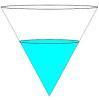Fill Me up Too

Age 14 to 16 Challenge Level:

In Fill Me Up we invited you to sketch graphs as vessels are filled with water. Can you work out the equations of the graphs?Air Routes

Age 16 to 18 Challenge Level:

Find the distance of the shortest air route at an altitude of 6000 metres between London and Cape Town given the latitudes and longitudes. A simple application of scalar products of vectors.Population Dynamics Collection

Age 16 to 18 Challenge Level:

This is our collection of tasks on the mathematical theme of 'Population Dynamics' for advanced students and those interested in mathematical modelling.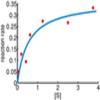Curve Fitter 2

Age 16 to 18 Challenge Level:

Can you construct a cubic equation with a certain distance between its turning points?Spherical Triangles on Very Big Spheres

Age 16 to 18 Challenge Level:

Shows that Pythagoras for Spherical Triangles reduces to Pythagoras's Theorem in the plane when the triangles are small relative to the radius of the sphere.Immersion

Age 14 to 16 Challenge Level:

Various solids are lowered into a beaker of water. How does the water level rise in each case?Over-booking

Age 16 to 18 Challenge Level:

The probability that a passenger books a flight and does not turn up is 0.05. For an aeroplane with 400 seats how many tickets can be sold so that only 1% of flights are over-booked?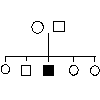Genetics

Age 14 to 16 Challenge Level:

A problem about genetics and the transmission of disease.Far Horizon

Age 14 to 16 Challenge Level:

An observer is on top of a lighthouse. How far from the foot of the lighthouse is the horizon that the observer can see?Stats Statements

Age 16 to 18 Challenge Level:

Are these statistical statements sometimes, always or never true? Or it is impossible to say?Taking Trigonometry Series-ly

Age 16 to 18 Challenge Level:

Look at the advanced way of viewing sin and cos through their power series.Bessel's Equation

Age 16 to 18 Challenge Level:

Get further into power series using the fascinating Bessel's equation.Time to Evolve 2

Age 16 to 18 Challenge Level:

How is the length of time between the birth of an animal and the birth of its great great ... great grandparent distributed?Scale Invariance

Age 16 to 18 Challenge Level:

By exploring the concept of scale invariance, find the probability that a random piece of real data begins with a 1.Real-life Equations

Age 16 to 18 Challenge Level:

Here are several equations from real life. Can you work out which measurements are possible from each equation?Designing Table Mats

Age 11 to 16 Challenge Level:

Formulate and investigate a simple mathematical model for the design of a table mat.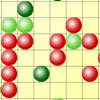Epidemic Modelling

Age 14 to 18 Challenge Level:

Use the computer to model an epidemic. Try out public health policies to control the spread of the epidemic, to minimise the number of sick days and deaths.